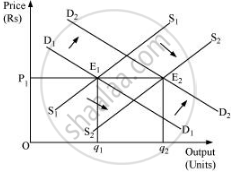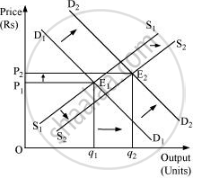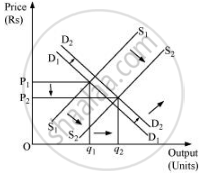Advertisement Remove all ads

# Explain Through a Diagram the Effect of a Rightward Shift of Both the Demand and Supply Curves on Equilibrium Price and Quantity. - Economics

Answer in Brief

Explain through a diagram the effect of a rightward shift of both the demand and supply curves on equilibrium price and quantity.

Advertisement Remove all ads

#### Solution

(a) When demand and supply increase in the same proportion:E1 is the initial equilibrium with equilibrium price P1 and equilibrium output q1.

Now, let us suppose that the demand increases to D2D2 and the supply increase to S2S2 by the same proportion. The new demand and new supply curve intersect at E2, which is the new equilibrium, with a new equilibrium output q2, but the same equilibrium price P1. Thus, an increase in the demand and the supply by the same proportion leaves the equilibrium price unchanged.

(b) When demand increases more than the increase in supply:

The original demand and supply curves intersect each other at E1 with initial equilibrium price P1 and initial equilibrium output q1.

Now, let us suppose that the demand increases and thereby the demand curve shifts to D2D2; the supply curve also shifts rightwards to S2S2. However, the increase in supply is less than the increase in demand. The new supply curve and the new demand curve intersect each other at point E2 with higher equilibrium price P2 and higher equilibrium output q2.(c) When the increase in demand is less than the increase in supply:

Let the initial equilibrium be at E1 with the equilibrium price P1 and equilibrium output q1. Now, let us suppose that the demand increases to D2D2 and the supply increases to S2S2; where the increase in supply is more than that of demand. The new demand curve D2D2 and the new supply curve S2S2 intersect at E2. Thus, the greater increase in supply curve as compared to the demand curve will lead the equilibrium price to fall and equilibrium output to rise.Concept: Market Supply Curve
Is there an error in this question or solution?
Advertisement Remove all ads

#### APPEARS IN

NCERT Class 12 Economics Introductory Microeconomics
Chapter 5 Market Equilibrium
Exercise | Q 15 | Page 87
Advertisement Remove all ads
Advertisement Remove all ads
Share
Notifications

View all notifications

Forgot password?